# Tag: Marcolli

Last time I mentioned the talk “From noncommutative geometry to the tropical geometry of the scaling site” by Alain Connes, culminating in the canonical isomorphism (last slide of the talk)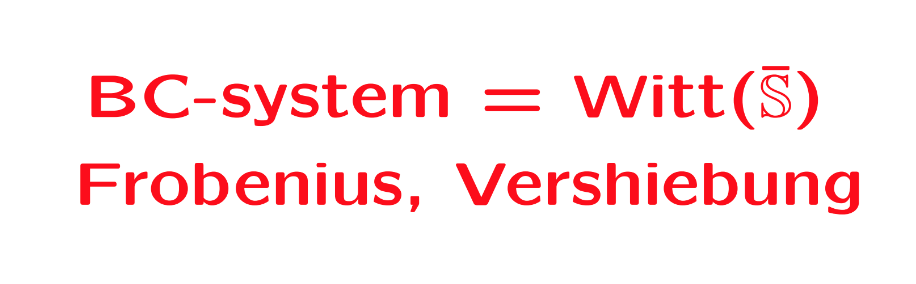Or rather, what is actually proved in his paper with Caterina Consani BC-system, absolute cyclotomy and the quantized calculus (and which they conjectured previously to be the case in Segal’s Gamma rings and universal arithmetic), is a canonical isomorphism between the $\lambda$-rings
$\mathbb{Z}[\mathbb{Q}/\mathbb{Z}] \simeq \mathbb{W}_0(\overline{\mathbb{S}})$
The left hand side is the integral groupring of the additive quotient-group $\mathbb{Q}/\mathbb{Z}$, or if you prefer, $\mathbb{Z}[\mathbf{\mu}_{\infty}]$ the integral groupring of the multiplicative group of all roots of unity $\mathbf{\mu}_{\infty}$.

The power maps on $\mathbf{\mu}_{\infty}$ equip $\mathbb{Z}[\mathbf{\mu}_{\infty}]$ with a $\lambda$-ring structure, that is, a family of commuting endomorphisms $\sigma_n$ with $\sigma_n(\zeta) = \zeta^n$ for all $\zeta \in \mathbf{\mu}_{\infty}$, and a family of linear maps $\rho_n$ induced by requiring for all $\zeta \in \mathbf{\mu}_{\infty}$ that
$\rho_n(\zeta) = \sum_{\mu^n=\zeta} \mu$
The maps $\sigma_n$ and $\rho_n$ are used to construct an integral version of the Bost-Connes algebra describing the Bost-Connes sytem, a quantum statistical dynamical system.

On the right hand side, $\mathbb{S}$ is the sphere spectrum (an object from stable homotopy theory) and $\overline{\mathbb{S}}$ its ‘algebraic closure’, that is, adding all abstract roots of unity.

The ring $\mathbb{W}_0(\overline{\mathbb{S}})$ is a generalisation to the world of spectra of the Almkvist-ring $\mathbb{W}_0(R)$ defined for any commutative ring $R$, constructed from pairs $(E,f)$ where $E$ is a projective $R$-module of finite rank and $f$ an $R$-endomorphism on it. Addition and multiplication are coming from direct sums and tensor products of such pairs, with zero element the pair $(0,0)$ and unit element the pair $(R,1_R)$. The ring $\mathbb{W}_0(R)$ is then the quotient-ring obtained by dividing out the ideal consisting of all zero-pairs $(E,0)$.

The ring $\mathbb{W}_0(R)$ becomes a $\lambda$-ring via the Frobenius endomorphisms $F_n$ sending a pair $(E,f)$ to the pair $(E,f^n)$, and we also have a collection of linear maps on $\mathbb{W}_0(R)$, the ‘Verschiebung’-maps which send a pair $(E,f)$ to the pair $(E^{\oplus n},F)$ with
$F = \begin{bmatrix} 0 & 0 & 0 & \cdots & f \\ 1 & 0 & 0 & \cdots & 0 \\ 0 & 1 & 0 & \cdots & 0 \\ \vdots & \vdots & \vdots & & \vdots \\ 0 & 0 & 0 & \cdots & 1 \end{bmatrix}$
Connes and Consani define a notion of modules and their endomorphisms for $\mathbb{S}$ and $\overline{\mathbb{S}}$, allowing them to define in a similar way the rings $\mathbb{W}_0(\mathbb{S})$ and $\mathbb{W}_0(\overline{\mathbb{S}})$, with corresponding maps $F_n$ and $V_n$. They then establish an isomorphism with $\mathbb{Z}[\mathbb{Q}/\mathbb{Z}]$ such that the maps $(F_n,V_n)$ correspond to $(\sigma_n,\rho_n)$.

But, do we really have the go to spectra to achieve this?

All this reminds me of an old idea of Yuri Manin mentioned in the introduction of his paper Cyclotomy and analytic geometry over $\mathbb{F}_1$, and later elaborated in section two of his paper with Matilde Marcolli Homotopy types and geometries below $\mathbf{Spec}(\mathbb{Z})$.

Take a manifold $M$ with a diffeomorphism $f$ and consider the corresponding discrete dynamical system by iterating the diffeomorphism. In such situations it is important to investigate the periodic orbits, or the fix-points $Fix(M,f^n)$ for all $n$. If we are in a situation that the number of fixed points is finite we can package these numbers in the Artin-Mazur zeta function
$\zeta_{AM}(M,f) = exp(\sum_{n=1}^{\infty} \frac{\# Fix(M,f^n)}{n}t^n)$
and investigate the properties of this function.

To connect this type of problem to Almkvist-like rings, Manin considers the Morse-Smale dynamical systems, a structural stable diffeomorphism $f$, having a finite number of non-wandering points on a compact manifold $M$.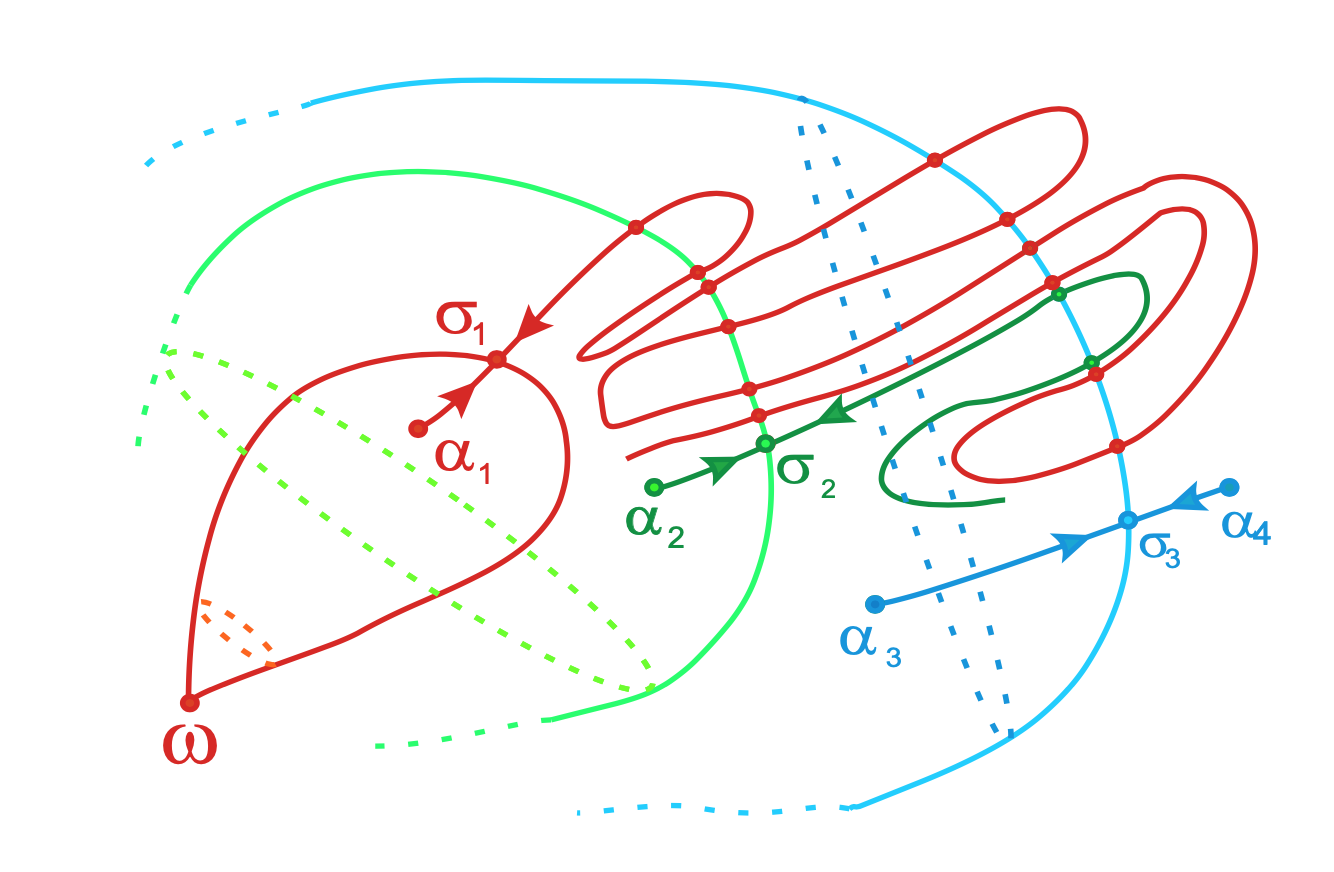From Topological classification of Morse-Smale diffeomorphisms on 3-manifolds

In such a situation $f_{\ast}$ acts on homology $H_k(M,\mathbb{Z})$, which are free $\mathbb{Z}$-modules of finite rank, as a matrix $M_f$ having only roots of unity as its eigenvalues.

Manin argues that this action is similar to the action of the Frobenius on etale cohomology groups, in which case the eigenvalues are Weil numbers. That is, one might view roots of unity as Weil numbers in characteristic one.

Clearly, all relevant data $(H_k(M,\mathbb{Z}),f_{\ast})$ belongs to the $\lambda$-subring of $\mathbb{W}_0(\mathbb{Z})$ generated by all pairs $(E,f)$ such that $M_f$ is diagonalisable and all its eigenvalues are either $0$ or roots of unity.

If we denote for any ring $R$ by $\mathbb{W}_1(R)$ this $\lambda$-subring of $\mathbb{W}_0(R)$, probably one would obtain canonical isomorphisms

– between $\mathbb{W}_1(\mathbb{Z})$ and the invariant part of the integral groupring $\mathbb{Z}[\mathbb{Q}/\mathbb{Z}]$ for the action of the group $Aut(\mathbb{Q}/\mathbb{Z}) = \widehat{\mathbb{Z}}^*$, and

– between $\mathbb{Z}[\mathbb{Q}/\mathbb{Z}]$ and $\mathbb{W}_1(\mathbb{Z}(\mathbf{\mu}_{\infty}))$ where $\mathbb{Z}(\mathbf{\mu}_{\infty})$ is the ring obtained by adjoining to $\mathbb{Z}$ all roots of unity.

A Belyi-extender (or dessinflateur) is a rational function $q(t) = \frac{f(t)}{g(t)} \in \mathbb{Q}(t)$ that defines a map
$q : \mathbb{P}^1_{\mathbb{C}} \rightarrow \mathbb{P}^1_{\mathbb{C}}$
unramified outside $\{ 0,1,\infty \}$, and has the property that $q(\{ 0,1,\infty \}) \subseteq \{ 0,1,\infty \}$.

An example of such a Belyi-extender is the power map $q(t)=t^n$, which is totally ramified in $0$ and $\infty$ and we clearly have that $q(0)=0,~q(1)=1$ and $q(\infty)=\infty$.

The composition of two Belyi-extenders is again an extender, and we get a rather mysterious monoid $\mathcal{E}$ of all Belyi-extenders.

Very little seems to be known about this monoid. Its units form the symmetric group $S_3$ which is the automrphism group of $\mathbb{P}^1_{\mathbb{C}} – \{ 0,1,\infty \}$, and mapping an extender $q$ to its degree gives a monoid map $\mathcal{E} \rightarrow \mathbb{N}_+^{\times}$ to the multiplicative monoid of positive natural numbers.

If one relaxes the condition of $q(t) \in \mathbb{Q}(t)$ to being defined over its algebraic closure $\overline{\mathbb{Q}}$, then such maps/functions have been known for some time under the name of dynamical Belyi-functions, for example in Zvonkin’s Belyi Functions: Examples, Properties, and Applications (section 6).

Here, one is interested in the complex dynamical system of iterations of $q$, that is, the limit-behaviour of the orbits
$\{ z,q(z),q^2(z),q^3(z),… \}$
for all complex numbers $z \in \mathbb{C}$.

In general, the 2-sphere $\mathbb{P}^1_{\mathbb{C}} = S^2$ has a finite number of open sets (the Fatou domains) where the limit behaviour of the series is similar, and the union of these open sets is dense in $S^2$. The complement of the Fatou domains is the Julia set of the function, of which we might expect a nice fractal picture.

Let’s take again the power map $q(t)=t^n$. For a complex number $z$ lying outside the unit disc, the series $\{ z,z^n,z^{2n},… \}$ has limit point $\infty$ and for those lying inside the unit circle, this limit is $0$. So, here we have two Fatou domains (interior and exterior of the unit circle) and the Julia set of the power map is the (boring?) unit circle.

Fortunately, there are indeed dynamical Belyi-maps having a more pleasant looking Julia set, such as this one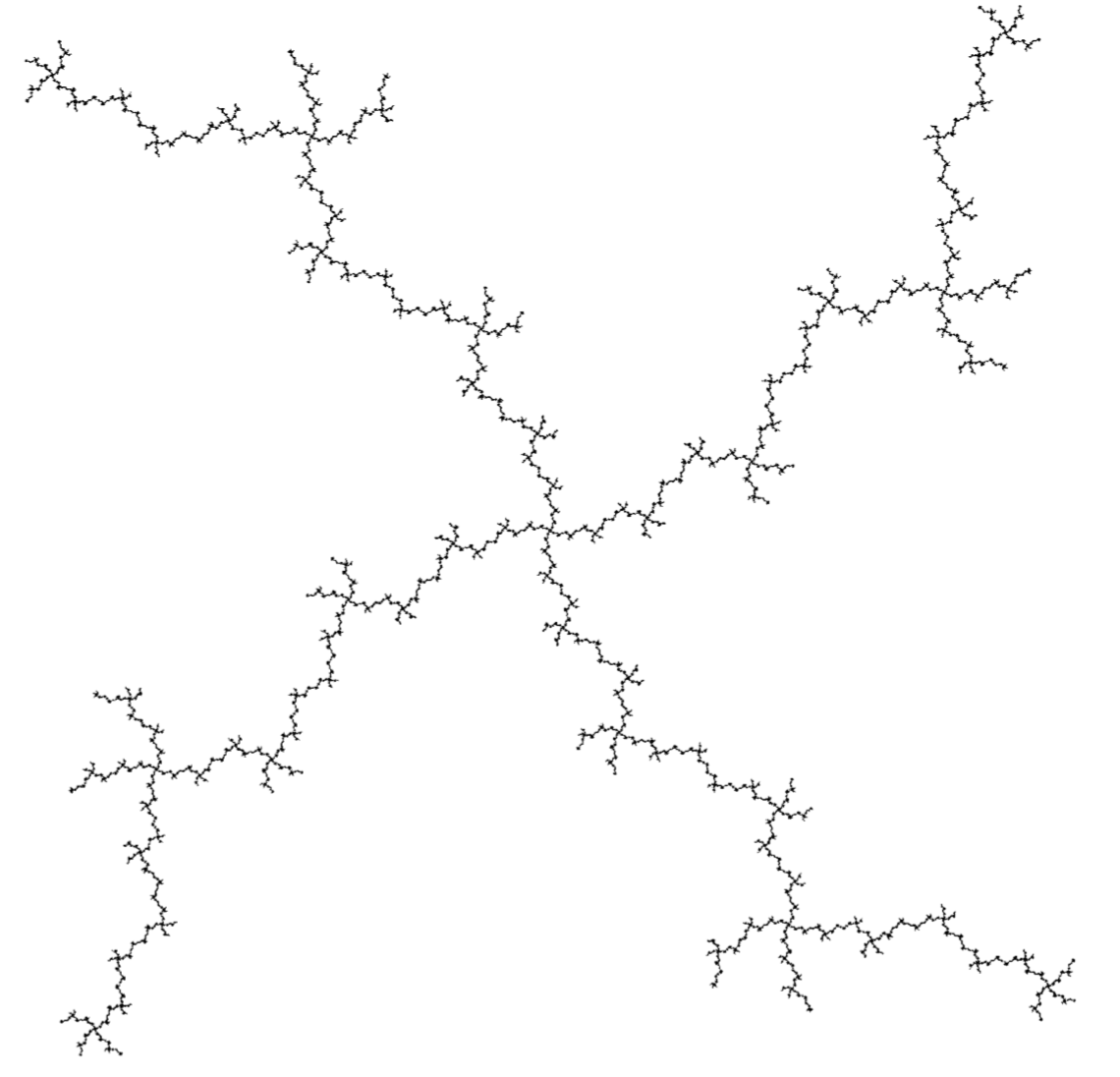But then, many dynamical Belyi-maps (and Belyi-extenders) are systems of an entirely different nature, they are completely chaotic, meaning that their Julia set is the whole $2$-sphere! Nowhere do we find an open region where points share the same limit behaviour… (the butterfly effect).

There’s a nice sufficient condition for chaotic behaviour, due to Dennis Sullivan, which is pretty easy to check for dynamical Belyi-maps.

A periodic point for $q(t)$ is a point $p \in S^2 = \mathbb{P}^1_{\mathbb{C}}$ such that $p = q^m(p)$ for some $m > 1$. A critical point is one such that either $q(p) = \infty$ or $q'(p)=0$.

Sullivan’s result is that $q(t)$ is completely chaotic when all its critical points $p$ become eventually periodic, that is some $q^k(p)$ is periodic, but $p$ itself is not periodic.

For a Belyi-map $q(t)$ the critical points are either comlex numbers mapping to $\infty$ or the inverse images of $0$ or $1$ (that is, the black or white dots in the dessin of $q(t)$) which are not leaf-vertices of the dessin.

Let’s do an example, already used by Sullivan himself:
$q(t) = (\frac{t-2}{t})^2$
This is a Belyi-function, and in fact a Belyi-extender as it is defined over $\mathbb{Q}$ and we have that $q(0)=\infty$, $q(1)=1$ and $q(\infty)=1$. The corresponding dessin is (inverse images of $\infty$ are marked with an $\ast$)The critical points $0$ and $2$ are not periodic, but they become eventually periodic:

$2 \rightarrow^q 0 \rightarrow^q \infty \rightarrow^q 1 \rightarrow^q 1$
and $1$ is periodic.

For a general Belyi-extender $q$, we have that the image under $q$ of any critical point is among $\{ 0,1,\infty \}$ and because we demand that $q(\{ 0,1,\infty \}) \subseteq \{ 0,1,\infty \}$, every critical point of $q$ eventually becomes periodic.

If we want to avoid the corresponding dynamical system to be completely chaotic, we have to ensure that one of the periodic points among $\{ 0,1,\infty \}$ (and there is at least one of those) must be critical.

Let’s consider the very special Belyi-extenders $q$ having the additional property that $q(0)=0$, $q(1)=1$ and $q(\infty)=\infty$, then all three of them are periodic.

So, the system is always completely chaotic unless the black dot at $0$ is not a leaf-vertex of the dessin, or the white dot at $1$ is not a leaf-vertex, or the degree of the region determined by the starred $\infty$ is at least two.

Going back to the mystery Manin-Marcolli sub-monoid of $\mathcal{E}$, it might explain why it is a good idea to restrict to very special Belyi-extenders having associated dessin a $2$-coloured tree, for then the periodic point $\infty$ is critical (the degree of the outside region is at least two), and therefore the conditions of Sullivan’s theorem are not satisfied. So, these Belyi-extenders do not necessarily have to be completely chaotic. (tbc)

A Belyi-extender (or dessinflateur) $\beta$ of degree $d$ is a quotient of two polynomials with rational coefficients
$\beta(t) = \frac{f(t)}{g(t)}$
with the special properties that for each complex number $c$ the polynomial equation of degree $d$ in $t$
$f(t)-c g(t)=0$
has $d$ distinct solutions, except perhaps for $c=0$ or $c=1$, and, in addition, we have that
$\beta(0),\beta(1),\beta(\infty) \in \{ 0,1,\infty \}$

Let’s take for instance the power maps $\beta_n(t)=t^n$.

For every $c$ the degree $n$ polynomial $t^n – c = 0$ has exactly $n$ distinct solutions, except for $c=0$, when there is just one. And, clearly we have that $0^n=0$, $1^n=1$ and $\infty^n=\infty$. So, $\beta_n$ is a Belyi-extender of degree $n$.

A cute observation being that if $\beta$ is a Belyi-extender of degree $d$, and $\beta’$ is an extender of degree $d’$, then $\beta \circ \beta’$ is again a Belyi-extender, this time of degree $d.d’$.

That is, Belyi-extenders form a monoid under composition!

In our example, $\beta_n \circ \beta_m = \beta_{n.m}$. So, the power-maps are a sub-monoid of the Belyi-extenders, isomorphic to the multiplicative monoid $\mathbb{N}_{\times}$ of strictly positive natural numbers.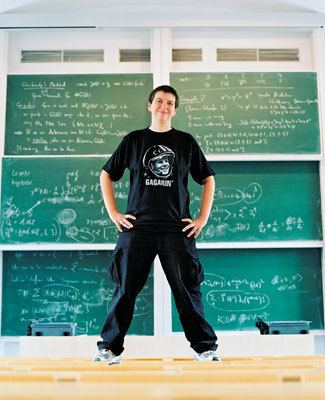In their paper Quantum statistical mechanics of the absolute Galois group, Yuri I. Manin and Matilde Marcolli say they use the full monoid of Belyi-extenders to act on all Grothendieck’s dessins d’enfant.

But, they attach properties to these Belyi-extenders which they don’t have, in general. That’s fine, as they foresee in Remark 2.21 of their paper that the construction works equally well for any suitable sub-monoid, as long as this sub-monoid contains all power-map exenders.

I’m trying to figure out what the maximal mystery sub-monoid of extenders is satisfying all the properties they need for their proofs.

But first, let us see what Belyi-extenders have to do with dessins d’enfant.In his user-friendlier period, Grothendieck told us how to draw a picture, which he called a dessin d’enfant, of an extender $\beta(t) = \frac{f(t)}{g(t)}$ of degree $d$:

Look at all complex solutions of $f(t)=0$ and label them with a black dot (and add a black dot at $\infty$ if $\beta(\infty)=0$). Now, look at all complex solutions of $f(t)-g(t)=0$ and label them with a white dot (and add a white dot at $\infty$ if $\beta(\infty)=1$).

Now comes the fun part.

Because $\beta$ has exactly $d$ pre-images for all real numbers $\lambda$ in the open interval $(0,1)$ (and $\beta$ is continuous), we can connect the black dots with the white dots by $d$ edges (the pre-images of the open interval $(0,1)$), giving us a $2$-coloured graph.

For the power-maps $\beta_n(t)=t^n$, we have just one black dot at $0$ (being the only solution of $t^n=0$), and $n$ white dots at the $n$-th roots of unity (the solutions of $x^n-1=0$). Any $\lambda \in (0,1)$ has as its $n$ pre-images the numbers $\zeta_i.\sqrt[n]{\lambda}$ with $\zeta_i$ an $n$-th root of unity, so we get here as picture an $n$-star. Here for $n=5$: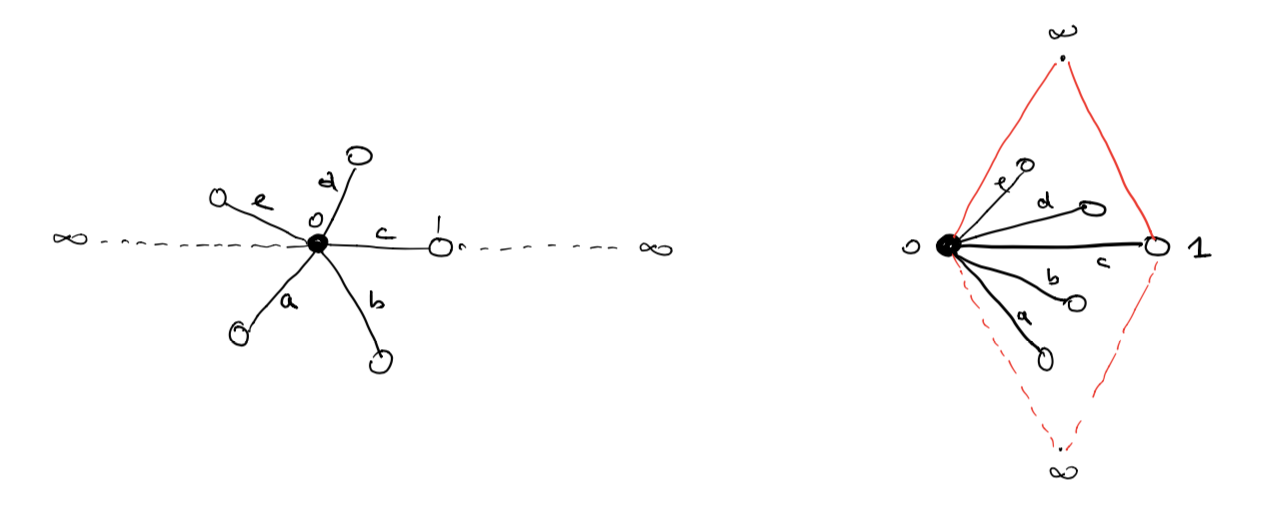This dessin should be viewed on the 2-sphere, with the antipodal point of $0$ being $\infty$, so projecting from $\infty$ gives a homeomorphism between the 2-sphere and $\mathbb{C} \cup \{ \infty \}$.

To get all information of the dessin (including possible dots at infinity) it is best to slice the sphere open along the real segments $(\infty,0)$ and $(1,\infty)$ and flatten it to form a ‘diamond’ with the upper triangle corresponding to the closed upper semisphere and the lower triangle to the open lower semisphere.

In the picture above, the right hand side is the dessin drawn in the diamond, and this representation will be important when we come to the action of extenders on more general Grothendieck dessins d’enfant.

Okay, let’s try to get some information about the monoid $\mathcal{E}$ of all Belyi-extenders.

What are its invertible elements?

Well, we’ve seen that the degree of a composition of two extenders is the product of their degrees, so invertible elements must have degree $1$, so are automorphisms of $\mathbb{P}^1_{\mathbb{C}} – \{ 0,1,\infty \} = S^2-\{ 0,1,\infty \}$ permuting the set $\{ 0,1,\infty \}$.

They form the symmetric group $S_3$ on $3$-letters and correspond to the Belyi-extenders
$t,~1-t,~\frac{1}{t},~\frac{1}{1-t},~\frac{t-1}{t},~\frac{t}{t-1}$
You can compose these units with an extender to get anther extender of the same degree where the roles of $0,1$ and $\infty$ are changed.

For example, if you want to colour all your white dots black and the black dots white, you compose with the unit $1-t$.

Manin and Marcolli use this and claim that you can transform any extender $\eta$ to an extender $\gamma$ by composing with a unit, such that $\gamma(0)=0, \gamma(1)=1$ and $\gamma(\infty)=\infty$.

That’s fine as long as your original extender $\eta$ maps $\{ 0,1,\infty \}$ onto $\{ 0,1,\infty \}$, but usually a Belyi-extender only maps into $\{ 0,1,\infty \}$.

Here are some extenders of degree three (taken from Melanie Wood’s paper Belyi-extending maps and the Galois action on dessins d’enfants):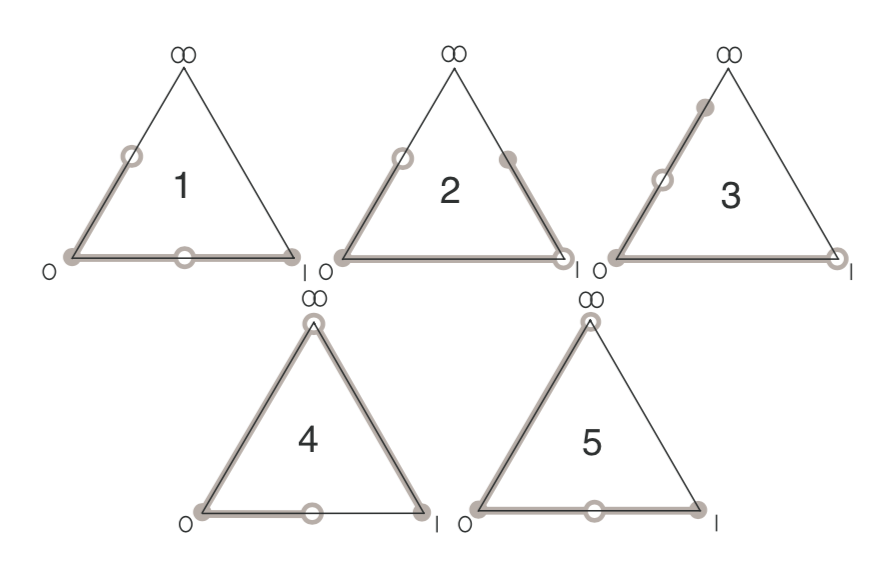with dessin $5$ corresponding to the Belyi-extender
$\beta(t) = \frac{t^2(t-1)}{(t-\frac{4}{3})^3}$
with $\beta(0)=0=\beta(1)$ and $\beta(\infty) = 1$.

So, a first property of the mystery Manin-Marcolli monoid $\mathcal{E}_{MMM}$ must surely be that all its elements $\gamma(t)$ map $\{ 0,1,\infty \}$ onto $\{ 0,1,\infty \}$, for they use this property a number of times, for instance to construct a monoid map
$\mathcal{E}_{MMM} \rightarrow M_2(\mathbb{Z})^+ \qquad \gamma \mapsto \begin{bmatrix} d & m-1 \\ 0 & 1 \end{bmatrix}$
where $d$ is the degree of $\gamma$ and $m$ is the number of black dots in the dessin (or white dots for that matter).

Further, they seem to believe that the dessin of any Belyi-extender must be a 2-coloured tree.

Already last time we’ve encountered a Belyi-extender $\zeta(t) = \frac{27 t^2(t-1)^2}{4(t^2-t+1)^3}$ with dessin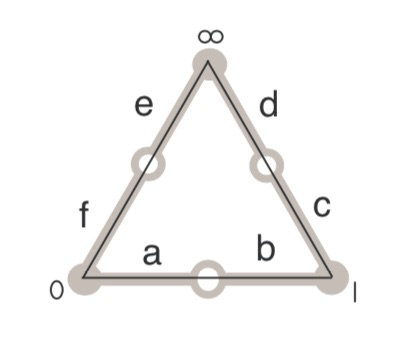But then, you may argue, this extender sends all of $0,1$ and $\infty$ to $0$, so it cannot belong to $\mathcal{E}_{MMM}$.

Here’s a trick to construct Belyi-extenders from Belyi-maps $\beta : \mathbb{P}^1 \rightarrow \mathbb{P}^1$, defined over $\mathbb{Q}$ and having the property that there are rational points in the fibers over $0,1$ and $\infty$.

Let’s take an example, the ‘monstrous dessin’ corresponding to the congruence subgroup $\Gamma_0(2)$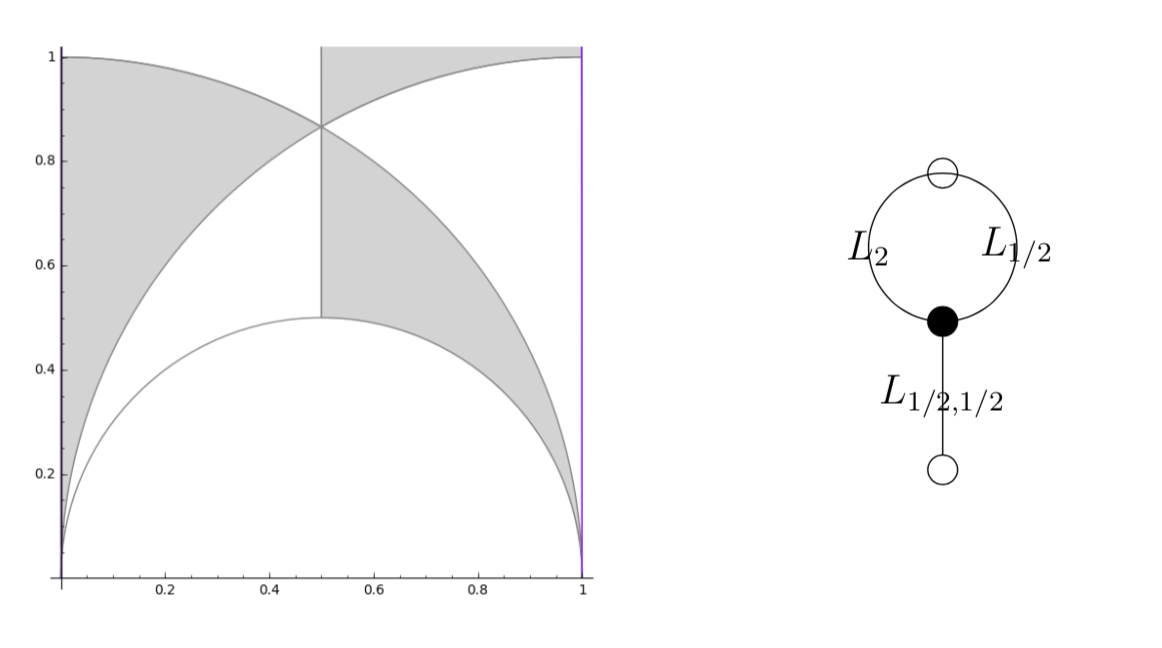with map $\beta(t) = \frac{(t+256)^3}{1728 t^2}$.

As it stands, $\beta$ is not a Belyi-extender because it does not map $1$ into $\{ 0,1,\infty \}$. But we have that
$-256 \in \beta^{-1}(0),~\infty \in \beta^{-1}(\infty),~\text{and}~512,-64 \in \beta^{-1}(1)$
(the last one follows from $(t+256)^2-1728 t^3=(t-512)^2(t+64)$).

We can now pre-compose $\beta$ with the automorphism (defined over $\mathbb{Q}$) sending $0$ to $-256$, $1$ to $-64$ and fixing $\infty$ to get a Belyi-extender
$\gamma(t) = \frac{(192t)^3}{1728(192t-256)^2}$
which maps $\gamma(0)=0,~\gamma(1)=1$ and $\gamma(\infty)=\infty$ (so belongs to $\mathcal{E}_{MMM}$) with the same dessin, which is not a tree,

That is, $\mathcal{E}_{MMM}$ can at best consist only of those Belyi-extenders $\gamma(t)$ that map $\{ 0,1,\infty \}$ onto $\{ 0,1,\infty \}$ and such that their dessin is a tree.

Let me stop, for now, by asking for a reference (or counterexample) to perhaps the most startling claim in the Manin-Marcolli paper, namely that any 2-coloured tree can be realised as the dessin of a Belyi-extender!

I’m trying to get into the latest Manin-Marcolli paper Quantum Statistical Mechanics of the Absolute Galois Group on how to create from Grothendieck’s dessins d’enfant a quantum system, generalising the Bost-Connes system to the non-Abelian part of the absolute Galois group $Gal(\overline{\mathbb{Q}}/\mathbb{Q})$.

In doing so they want to extend the action of the multiplicative monoid $\mathbb{N}_{\times}$ by power maps on the roots of unity to the action of a larger monoid on all dessins d’enfants.

Here they use an idea, originally due to Jordan Ellenberg, worked out by Melanie Wood in her paper Belyi-extending maps and the Galois action on dessins d’enfants.To grasp this, it’s best to remember what dessins have to do with Belyi maps, which are maps defined over $\overline{\mathbb{Q}}$
$\pi : \Sigma \rightarrow \mathbb{P}^1$
from a Riemann surface $\Sigma$ to the complex projective line (aka the 2-sphere), ramified only in $0,1$ and $\infty$. The dessin determining $\pi$ is the 2-coloured graph on the surface $\Sigma$ with as black vertices the pre-images of $0$, white vertices the pre-images of $1$ and these vertices are joined by the lifts of the closed interval $[0,1]$, so the number of edges is equal to the degree $d$ of the map.

Wood considers a very special subclass of these maps, which she calls Belyi-extender maps, of the form
$\gamma : \mathbb{P}^1 \rightarrow \mathbb{P}^1$
defined over $\mathbb{Q}$ with the additional property that $\gamma$ maps $\{ 0,1,\infty \}$ into $\{ 0,1,\infty \}$.

The upshot being that post-compositions of Belyi’s with Belyi-extenders $\gamma \circ \pi$ are again Belyi maps, and if two Belyi’s $\pi$ and $\pi’$ lie in the same Galois orbit, then so must all $\gamma \circ \pi$ and $\gamma \circ \pi’$.

The crucial Ellenberg-Wood idea is then to construct “new Galois invariants” of dessins by checking existing and easily computable Galois invariants on the dessins of the Belyi’s $\gamma \circ \pi$.

For this we need to know how to draw the dessin of $\gamma \circ \pi$ on $\Sigma$ if we know the dessins of $\pi$ and of the Belyi-extender $\gamma$. Here’s the procedure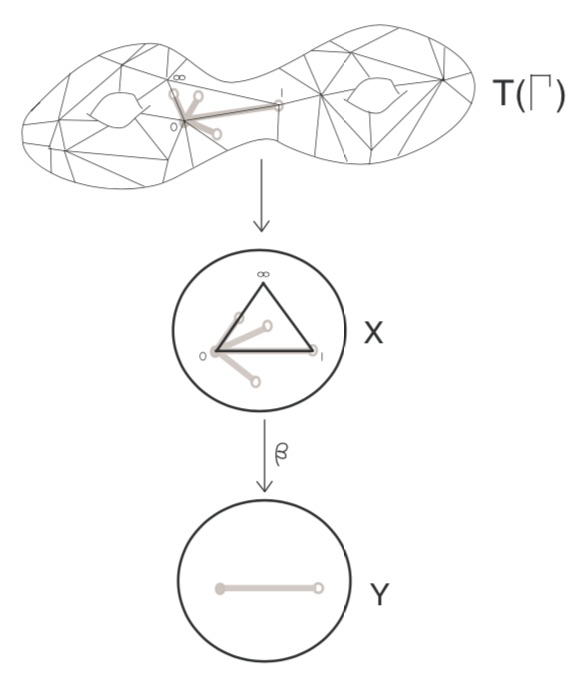Here, the middle dessin is that of the Belyi-extender $\gamma$ (which in this case is the power map $t \rightarrow t^4$) and the upper graph is the unmarked dessin of $\pi$.

One has to replace each of the black-white edges in the dessin of $\pi$ by the dessin of the expander $\gamma$, but one must be very careful in respecting the orientations on the two dessins. In the upper picture just one edge is replaced and one has to do this for all edges in a compatible manner.

Thus, a Belyi-expander $\gamma$ inflates the dessin $\pi$ with factor the degree of $\gamma$. For this reason i prefer to call them dessinflateurs, a contraction of dessin+inflator.

In her paper, Melanie Wood says she can separate dessins for which all known Galois invariants were the same, such as these two dessins,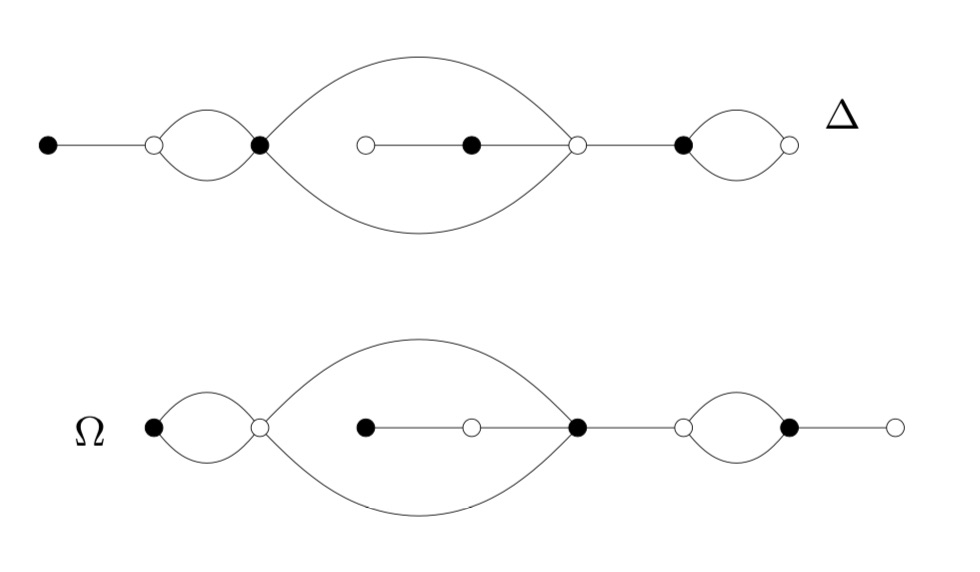by inflating them with a suitable Belyi-extender and computing the monodromy group of the inflated dessin.

This monodromy group is the permutation group generated by two elements, the first one gives the permutation on the edges given by walking counter-clockwise around all black vertices, the second by walking around all white vertices.

For example, by labelling the edges of $\Delta$, its monodromy is generated by the permutations $(2,3,5,4)(1,6)(8,10,9)$ and $(1,3,2)(4,7,5,8)(9,10)$ and GAP tells us that the order of this group is $1814400$. For $\Omega$ the generating permutations are $(1,2)(3,6,4,7)(8,9,10)$ and $(1,2,4,3)(5,6)(7,9,8)$, giving an isomorphic group.

Let’s inflate these dessins using the Belyi-extender $\gamma(t) = -\frac{27}{4}(t^3-t^2)$ with corresponding dessin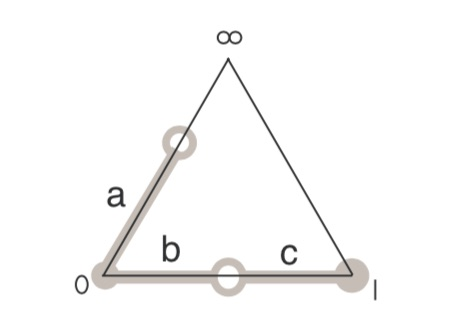It took me a couple of attempts before I got the inflated dessins correct (as i knew from Wood that this simple extender would not separate the dessins). Inflated $\Omega$ on top: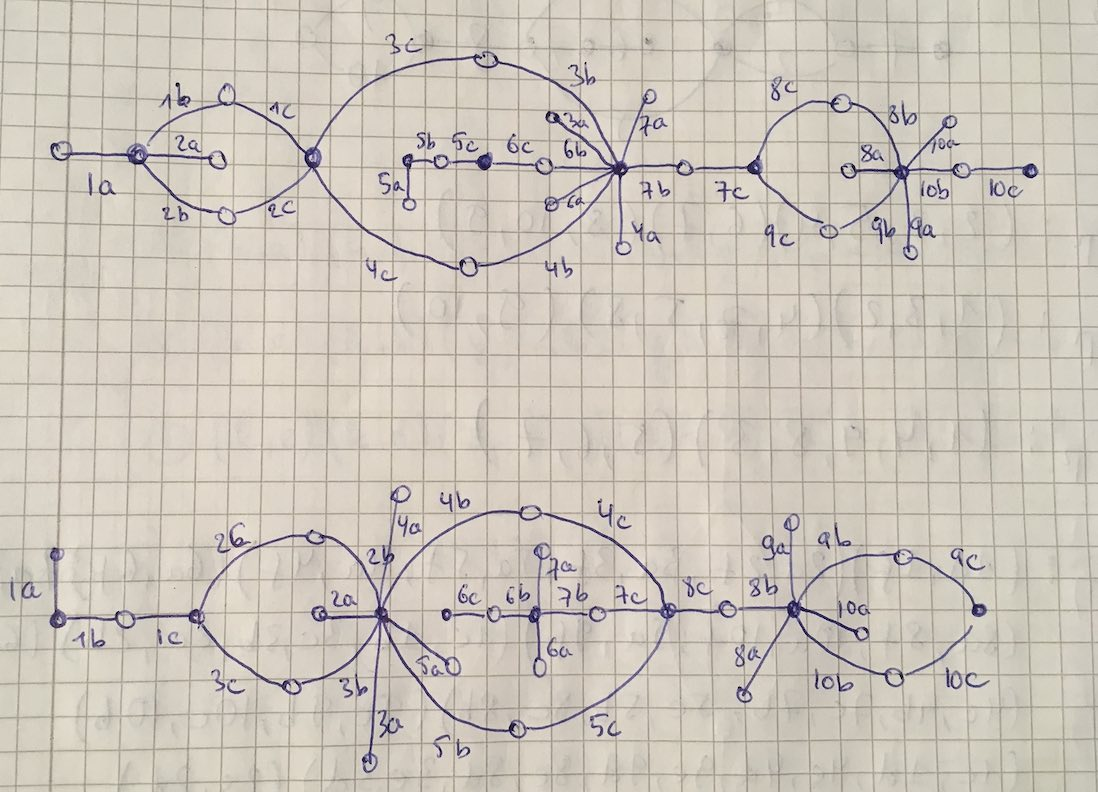Both dessins give a monodromy group of order $35838544379904000000$.

Now we’re ready to do serious work.

Melanie Wood uses in her paper the extender $\zeta(t)=\frac{27 t^2(t-1)^2}{4(t^2-t+1)^3}$ with associated dessinand says she can now separate the inflated dessins by the order of their monodromy groups. She gets for the inflated $\Delta$ the order $19752284160000$ and for inflated $\Omega$ the order $214066877211724763979841536000000000000$.

It’s very easy to make mistakes in these computations, so probably I did something horribly wrong but I get for both $\Delta$ and $\Omega$ that the order of the monodromy group of the inflated dessin is $214066877211724763979841536000000000000$.

I’d be very happy when someone would be able to spot the error!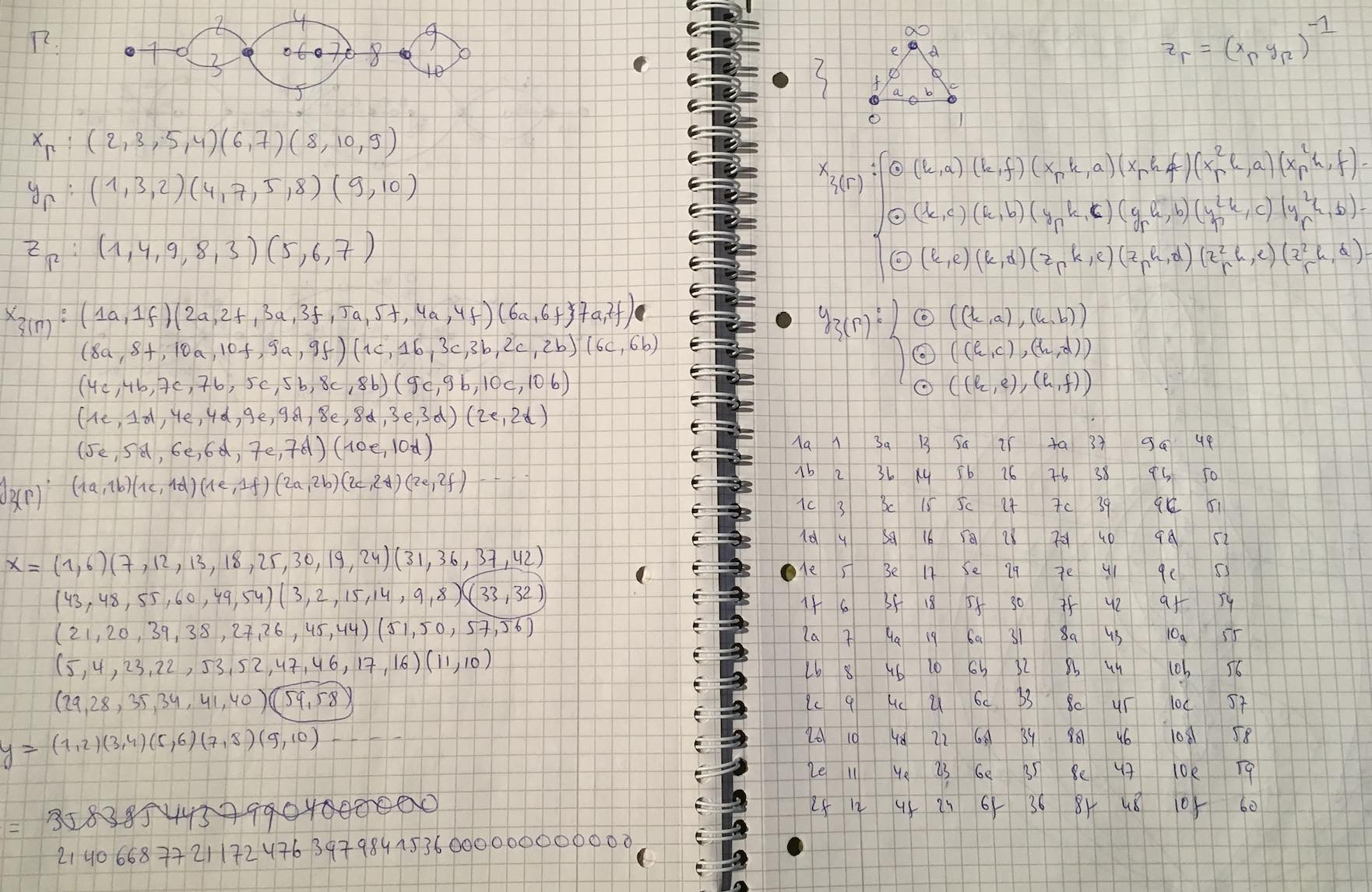The Bulletin of the AMS just made this paper by Julia Mueller available online: “On the genesis of Robert P. Langlands’ conjectures and his letter to Andre Weil” (hat tip +ChandanDalawat and +DavidRoberts on Google+).

It recounts the story of the early years of Langlands and the first years of his mathematical career (1960-1966)leading up to his letter to Andre Weil in which he outlines his conjectures, which would become known as the Langlands program.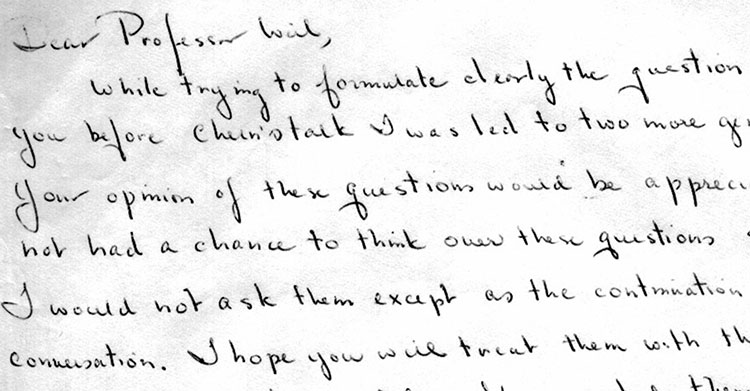Langlands letter to Weil is available from the IAS.

The Langlands program is a vast net of conjectures. For example, it conjectures that there is a correspondence between

– $n$-dimensional representations of the absolute Galois group $Gal(\overline{\mathbb{Q}}/\mathbb{Q})$, and

– specific data coming from an adelic quotient-space $GL_n(\mathbb{A}_{\mathbb{Q}})/GL_n(\mathbb{Q})$.

For $n=1$ this is essentially class field theory with the correspondence given by Artin’s reciprocity law.

Here we have on the one hand the characters of the abelianised absolute Galois group

$Gal(\overline{\mathbb{Q}}/\mathbb{Q})^{ab} \simeq Gal(\mathbb{Q}(\pmb{\mu}_{\infty})/\mathbb{Q}) \simeq \widehat{\mathbb{Z}}^{\ast}$

and on the other hand the connected components of the idele class space

$GL_1(\mathbb{A}_{\mathbb{Q}})/GL_1(\mathbb{Q}) = \mathbb{A}_{\mathbb{Q}}^{\ast} / \mathbb{Q}^{\ast} = \mathbb{R}_+^{\ast} \times \widehat{\mathbb{Z}}^{\ast}$

For $n=2$ it involves the study of Galois representations coming from elliptic curves. A gentle introduction to the general case is Mark Kisin’s paper What is … a Galois representation?.

One way to look at some of the quantum statistical systems studied via non-commutative geometry is that they try to understand the “bad” boundary of the Langlands space $GL_n(\mathbb{A}_{\mathbb{Q}})/GL_n(\mathbb{Q})$.

Here, the Bost-Connes system corresponds to the $n=1$ case, the Connes-Marcolli system to the $n=2$ case.

If $\mathbb{A}’_{\mathbb{Q}}$ is the subset of all adeles having almost all of its terms in $\widehat{\mathbb{Z}}_p^{\ast}$, then there is a well-defined map

$\pi~:~\mathbb{A}’_{\mathbb{Q}}/\mathbb{Q}^{\ast} \rightarrow \mathbb{R}_+ \qquad (x_{\infty},x_2,x_2,\dots) \mapsto | x_{\infty} | \prod_p | x_p |_p$

The inverse image of $\pi$ over $\mathbb{R}_+^{\ast}$ are exactly the idele classes $\mathbb{A}_{\mathbb{Q}}^{\ast}/\mathbb{Q}^{\ast}$, so we can view them as the nice locus of the horrible complicated quotient of adele-classes $\mathbb{A}_{\mathbb{Q}}/\mathbb{Q}^*$. And we can view the adele-classes as a ‘closure’ of the idele classes.

But, the fiber $\pi^{-1}(0)$ has horrible topological properties because $\mathbb{Q}^*$ acts ergodically on it due to the fact that $log(p)/log(q)$ is irrational for distinct primes $p$ and $q$.

This is why it is better to view the adele-classes not as an ordinary space (one with bad topological properties), but rather as a ‘non-commutative’ space because it is controlled by a non-commutative algebra, the Bost-Connes algebra.

For $n=2$ there’s a similar story with a ‘bad’ quotient $M_2(\mathbb{A}_{\mathbb{Q}})/GL_2(\mathbb{Q})$, being the closure of an ‘open’ nice piece which is the Langlands quotient space $GL_2(\mathbb{A}_{\mathbb{Q}})/GL_2(\mathbb{Q})$.

A well-known link between Conway’s Big Picture and non-commutative geometry is given by the Bost-Connes system.

This quantum statistical mechanical system encodes the arithmetic properties of cyclotomic extensions of $\mathbb{Q}$.

The corresponding Bost-Connes algebra encodes the action by the power-maps on the roots of unity.

It has generators $e_n$ and $e_n^*$ for every natural number $n$ and additional generators $e(\frac{g}{h})$ for every element in the additive group $\mathbb{Q}/\mathbb{Z}$ (which is of course isomorphic to the multiplicative group of roots of unity).

The defining equations are
$\begin{cases} e_n.e(\frac{g}{h}).e_n^* = \rho_n(e(\frac{g}{h})) \\ e_n^*.e(\frac{g}{h}) = \Psi^n(e(\frac{g}{h}).e_n^* \\ e(\frac{g}{h}).e_n = e_n.\Psi^n(e(\frac{g}{h})) \\ e_n.e_m=e_{nm} \\ e_n^*.e_m^* = e_{nm}^* \\ e_n.e_m^* = e_m^*.e_n~\quad~\text{if (m,n)=1} \end{cases}$

Here $\Psi^n$ are the power-maps, that is $\Psi^n(e(\frac{g}{h})) = e(\frac{ng}{h}~mod~1)$, and the maps $\rho_n$ are given by
$\rho_n(e(\frac{g}{h})) = \sum e(\frac{i}{j})$
where the sum is taken over all $\frac{i}{j} \in \mathbb{Q}/\mathbb{Z}$ such that $n.\frac{i}{j}=\frac{g}{h}$.

Conway’s Big Picture has as its vertices the (equivalence classes of) lattices $M,\frac{g}{h}$ with $M \in \mathbb{Q}_+$ and $\frac{g}{h} \in \mathbb{Q}/\mathbb{Z}$.

The Bost-Connes algebra acts on the vector-space with basis the vertices of the Big Picture. The action is given by:
$\begin{cases} e_n \ast \frac{c}{d},\frac{g}{h} = \frac{nc}{d},\rho^m(\frac{g}{h})~\quad~\text{with m=(n,d)} \\ e_n^* \ast \frac{c}{d},\frac{g}{h} = (n,c) \times \frac{c}{nd},\Psi^{\frac{n}{m}}(\frac{g}{h})~\quad~\text{with m=(n,c)} \\ e(\frac{a}{b}) \ast \frac{c}{d},\frac{g}{h} = \frac{c}{d},\Psi^c(\frac{a}{b}) \frac{g}{h} \end{cases}$

This connection makes one wonder whether non-commutative geometry can shed a new light on monstrous moonshine?

This question is taken up by Jorge Plazas in his paper Non-commutative geometry of groups like $\Gamma_0(N)$Plazas shows that the bigger Connes-Marcolli $GL_2$-system also acts on the Big Picture. An intriguing quote:

“Our interest in the $GL_2$-system comes from the fact that its thermodynamic properties encode the arithmetic theory of modular functions to an extend which makes it possible for us to capture aspects of moonshine theory.”

Looks like the right kind of paper to take along when I disappear next week for some time in the French mountains…

Last fall, Matilde Marcolli gave a course at CalTech entitled Oral Presentation: The (Martial) Art of Giving Talks. The purpose of this course was to teach students “how to effectively communicate their work in seminars and conferences and how to defend it from criticism from the audience”.

The lecture notes contain basic information on the different types of talks and how to prepare them. But they really shine when it comes to spotting the badasses in the public and how to respond to their interference. She identifies 5 badsass-types : the empreror and the hierophant (see below), the chariot (the one with a literal mind, asking continuously for details), the fool (the one who happens to sit in the talk but doesn’t belong there) and the magician (the quick smartass).

I’ll just quote here the description of, and most effective strategy against, the first two badass-types. Please have a look at the whole paper, it is a good read!“The Emperor is the typical figure of power and authority in a given field. It refers to those people who have a tendency to think that the whole field is their own private property, and in particular that only what they do in the field is important, that the work of all others is derivative and that in any case they are not being quoted enough. These are typically pathological narcissists, so one needs to take this into account in interacting with them.
The trouble of having The Emperor in your audience is that he (it is rarely she) can very easily disrupt your presentation completely, by continuous interruptions, by running his own commentary while you are trying to stay focused on delivering your talk and by distracting the rest of the audience.
The Emperor is by far one of the most dangerous encounters you can make in the wilderness of the conference rooms.”

Counter-measure : “Keep in mind that the Emperor is a pathological narcissist: part of the reason why he keeps interrupting your talk is because he cannot stand the fact that, during those fifty minutes, the attention of the audience is focused on you and not on him. His continuous interruptions and complaints are a way to try to divert the attention of the audience back to him and away from you. That your talk gets disrupted in the process, he could not care the less.
A good way to try to avoid the worst case scenario is to make sure (if you know in advance you may be having the Emperor in the audience) that you arrange in your talk to make frequent references to him and his work. In this way, he will hopefully feel that his need to be at the center of attention is sufficiently satisfied that he can let you continue with your talk. Effectiveness: high.”“The Hierophant represents a priestly figure. What this refers to here is the type of character who feels entitled to represent (and defend) a certain “orthodoxy”, a certain school of thought, or a certain group of people within the field.
Typically the hierophants are the minions and lackeys of the Emperor, his entourage and fan club, those who think that the Emperor represents the only and true orthodoxy in the field and that anything that is done in a different way should be opposed and suppressed.
These characters are generally less disruptive than the Emperor himself, as they are really only fighting you on someone else’s behalf. Nonetheless, they can sometime manage to seriously disrupt your presentation.”

Counter-measure : “This is essentially the same advise as in the case of the Emperor. To an objection that substantially is of the form: “This is not the right way to do things because this is not what what we do (= what the Emperor does)”, which is what you expect to hear from the Hierophant, you can reply along lines such as: “There is also another approach to this problem, developed by the Emperor and his school, which is a very interesting approach that gave nice and important results. However, this is not what I am talking about today: I am talking here about a different approach, and I will be focusing only on the specific features of this other approach…”
Something along these lines would recognize “their” work without having to make any concession on their approach being the only game in town.
Effectiveness: high (unless the Emperor is also present and is delegating to his hierophants the task of attacking you: in that case they won’t give up so easily and the effectiveness of this line of defense becomes medium/low).”

Previously, we have recalled comparisons between approaches to define a geometry over the absolute point and art-historical movements, first those due to Yuri I. Manin, subsequently some extra ones due to Javier Lopez Pena and Oliver Lorscheid.

In these comparisons, the art trend appears to have been chosen more to illustrate a key feature of the approach or an appreciation of its importance, rather than giving a visual illustration of the varieties over $\mathbb{F}_1$ the approach proposes.

Some time ago, we’ve had a couple of posts trying to depict noncommutative varieties, first the illustrations used by Shahn Majid and Matilde Marcolli, and next my own mental picture of it.

In this post, we’ll try to do something similar for affine varieties over the absolute point. To simplify things drastically, I’ll divide the islands in the Lopez Pena-Lorscheid map of $\mathbb{F}_1$ land in two subsets : the former approaches (all but the $\Lambda$-schemes) and the current approach (the $\Lambda$-scheme approach due to James Borger).

The former approaches : Francis Bacon “The Pope” (1953)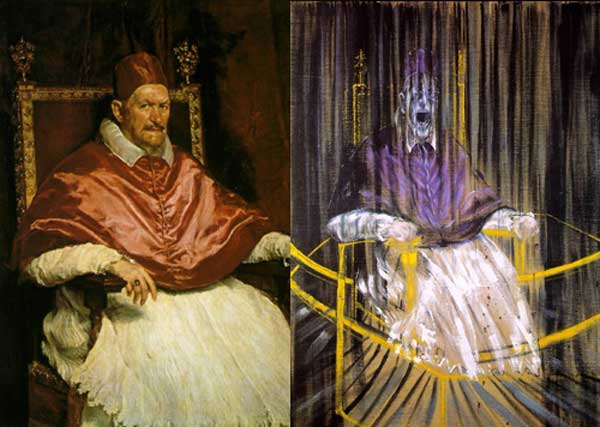The general consensus here was that in going from $\mathbb{Z}$ to $\mathbb{F}_1$ one looses the additive structure and retains only the multiplicative one. Hence, ‘commutative algebras’ over $\mathbb{F}_1$ are (commutative) monoids, and mimicking Grothendieck’s functor of points approach to algebraic geometry, a scheme over $\mathbb{F}_1$ would then correspond to a functor

$h_Z~:~\mathbf{monoids} \longrightarrow \mathbf{sets}$

Such functors are described largely by combinatorial data (see for example the recent blueprint-paper by Oliver Lorscheid), and, if the story would stop here, any Rothko painting could be used as illustration.

Most of the former approaches add something though (buzzwords include ‘Arakelov’, ‘completion at $\infty$’, ‘real place’ etc.) in order to connect the virtual geometric object over $\mathbb{F}_1$ with existing real, complex or integral schemes. For example, one can make the virtual object visible via an evaluation map $h_Z \rightarrow h_X$ which is a natural transformation, where $X$ is a complex variety with its usual functor of points $h_X$ and to connect both we associate to a monoid $M$ its complex monoid-algebra $\mathbb{C} M$. An integral scheme $Y$ can then be said to be ‘defined over $\mathbb{F}_1$’, if $h_Z$ becomes a subfunctor of its usual functor of points $h_Y$ (again, assigning to a monoid its integral monoid algebra $\mathbb{Z} M$) and $Y$ is the ‘best’ integral scheme approximation of the complex evaluation map.

To illustrate this, consider the painting Study after Velázquez’s Portrait of Pope Innocent X by Francis Bacon (right-hand painting above) which is a distorded version of the left-hand painting Portrait of Innocent X by Diego Velázquez.

Here, Velázquez’ painting plays the role of the complex variety which makes the combinatorial gadget $h_Z$ visible, and, Bacon’s painting depicts the integral scheme, build up from this combinatorial data, which approximates the evaluation map best.

All of the former approaches more or less give the same very small list of integral schemes defined over $\mathbb{F}_1$, none of them motivically interesting.

The current approach : Jackson Pollock “No. 8” (1949)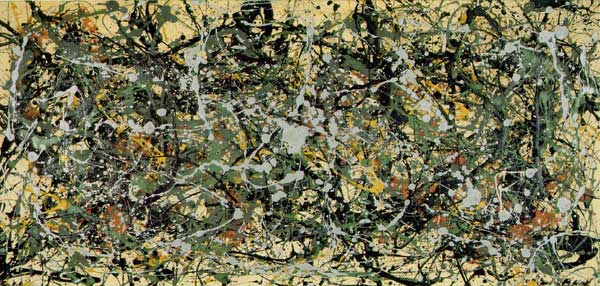An entirely different approach was proposed by James Borger in $\Lambda$-rings and the field with one element. He proposes another definition for commutative $\mathbb{F}_1$-algebras, namely $\lambda$-rings (in the sense of Grothendieck’s Riemann-Roch) and he argues that the $\lambda$-ring structure (which amounts in the sensible cases to a family of endomorphisms of the integral ring lifting the Frobenius morphisms) can be viewed as descent data from $\mathbb{Z}$ to $\mathbb{F}_1$.

The list of integral schemes of finite type with a $\lambda$-structure coincides roughly with the list of integral schemes defined over $\mathbb{F}_1$ in the other approaches, but Borger’s theory really shines in that it proposes long sought for mystery-objects such as $\mathbf{spec}(\mathbb{Z}) \times_{\mathbf{spec}(\mathbb{F}_1)} \mathbf{spec}(\mathbb{Z})$. If one accepts Borger’s premise, then this object should be the geometric object corresponding to the Witt-ring $W(\mathbb{Z})$. Recall that the role of Witt-rings in $\mathbb{F}_1$-geometry was anticipated by Manin in Cyclotomy and analytic geometry over $\mathbb{F}_1$.

But, Witt-rings and their associated Witt-spaces are huge objects, so one needs to extend arithmetic geometry drastically to include such ‘integral schemes of infinite type’. Borger has made a couple of steps in this direction in The basic geometry of Witt vectors, II: Spaces.

To depict these new infinite dimensional geometric objects I’ve chosen for Jackson Pollock‘s painting No. 8. It is no coincidence that Pollock-paintings also appeared in the depiction of noncommutative spaces. In fact, Matilde Marcolli has made the connection between $\lambda$-rings and noncommutative geometry in Cyclotomy and endomotives by showing that the Bost-Connes endomotives are universal for $\lambda$-rings.Last time we did recall Manin’s comparisons between some approaches to geometry over the absolute point $\pmb{spec}(\mathbb{F}_1)$ and trends in the history of art.

In the comments to that post, Javier Lopez-Pena wrote that he and Oliver Lorscheid briefly contemplated the idea of extending Manin’s artsy-dictionary to all approaches they did draw on their Map of $\mathbb{F}_1$-land.

So this time, we will include here Javier’s and Oliver’s insights on the colored pieces below in their map : CC=Connes-Consani, Generalized torified schemes=Lopez Pena-Lorscheid, Generalized schemes with 0=Durov and, this time, $\Lambda$=Manin-Marcolli.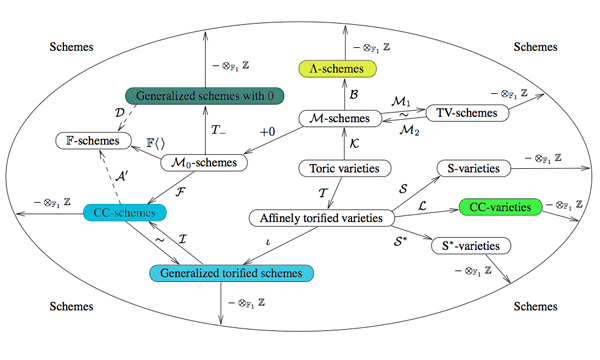Durov : romanticismIn his 568 page long Ph.D. thesis New Approach to Arakelov Geometry Nikolai Durov introduces a vast generalization of classical algebraic geometry in which both Arakelov geometry and a more exotic geometry over $\mathbb{F}_1$ fit naturally. Because there were great hopes and expectations it would lead to a big extension of algebraic geometry, Javier and Oliver associate this approach to romantism. From wikipedia : “The modern sense of a romantic character may be expressed in Byronic ideals of a gifted, perhaps misunderstood loner, creatively following the dictates of his inspiration rather than the standard ways of contemporary society.”

Manin and Marcolli : impressionismYuri I. Manin in Cyclotomy and analytic geometry over $\mathbb{F}_1$ and Matilde Marcolli in Cyclotomy and endomotives develop a theory of analytic geometry over $\mathbb{F}_1$ based on analytic functions ‘leaking out of roots of unity’. Javier and Oliver depict such functions as ‘thin, but visible brush strokes at roots of 1’ and therefore associate this approach to impressionism. Frow wikipedia : ‘Characteristics of Impressionist paintings include: relatively small, thin, yet visible brush strokes; open composition; emphasis on accurate depiction of light in its changing qualities (often accentuating the effects of the passage of time); common, ordinary subject matter; the inclusion of movement as a crucial element of human perception and experience; and unusual visual angles.’

Connes and Consani : cubismIn On the notion of geometry over $\mathbb{F}_1$ Alain Connes and Katia Consani develop their extension of Soule’s approach. A while ago I’ve done a couple of posts on this here, here and here. Javier and Oliver associate this approach to cubism (a.o. Pablo Picasso and Georges Braque) because of the weird juxtapositions of the simple monoidal pieces in this approach.

Lopez-Pena and Lorscheid : deconstructivismTorified varieties and schemes were introduced by Javier Lopez-Pena and Oliver Lorscheid in Torified varieties and their geometries over $\mathbb{F}_1$ to get lots of examples of varieties over the absolute point in the sense of both Soule and Connes-Consani. Because they were fragmenting schemes into their “fundamental pieces” they associate their approach to deconstructivism.

Another time I’ll sketch my own arty-farty take on all this.

This is a belated response to a Math-Overflow exchange between Thomas Riepe and Chandan Singh Dalawat asking for a possible connection between Connes’ noncommutative geometry approach to the Riemann hypothesis and the Langlands program.

Here’s the punchline : a large chunk of the Connes-Marcolli book Noncommutative Geometry, Quantum Fields and Motives can be read as an exploration of the noncommutative boundary to the Langlands program (at least for $GL_1$ and $GL_2$ over the rationals $\mathbb{Q}$).

Recall that Langlands for $GL_1$ over the rationals is the correspondence, given by the Artin reciprocity law, between on the one hand the abelianized absolute Galois group

$Gal(\overline{\mathbb{Q}}/\mathbb{Q})^{ab} = Gal(\mathbb{Q}(\mu_{\infty})/\mathbb{Q}) \simeq \hat{\mathbb{Z}}^*$

and on the other hand the connected components of the idele classes

$\mathbb{A}^{\ast}_{\mathbb{Q}}/\mathbb{Q}^{\ast} = \mathbb{R}^{\ast}_{+} \times \hat{\mathbb{Z}}^{\ast}$

The locally compact Abelian group of idele classes can be viewed as the nice locus of the horrible quotient space of adele classes $\mathbb{A}_{\mathbb{Q}}/\mathbb{Q}^{\ast}$. There is a well-defined map

$\mathbb{A}_{\mathbb{Q}}’/\mathbb{Q}^{\ast} \rightarrow \mathbb{R}_{+} \qquad (x_{\infty},x_2,x_3,\ldots) \mapsto | x_{\infty} | \prod | x_p |_p$

from the subset $\mathbb{A}_{\mathbb{Q}}’$ consisting of adeles of which almost all terms belong to $\mathbb{Z}_p^{\ast}$. The inverse image of this map over $\mathbb{R}_+^{\ast}$ are precisely the idele classes $\mathbb{A}^{\ast}_{\mathbb{Q}}/\mathbb{Q}^{\ast}$. In this way one can view the adele classes as a closure, or ‘compactification’, of the idele classes.

This is somewhat reminiscent of extending the nice action of the modular group on the upper-half plane to its badly behaved action on the boundary as in the Manin-Marcolli cave post.

The topological properties of the fiber over zero, and indeed of the total space of adele classes, are horrible in the sense that the discrete group $\mathbb{Q}^*$ acts ergodically on it, due to the irrationality of $log(p_1)/log(p_2)$ for primes $p_i$. All this is explained well (in the semi-local case, that is using $\mathbb{A}_Q’$ above) in the Connes-Marcolli book (section 2.7).

In much the same spirit as non-free actions of reductive groups on algebraic varieties are best handled using stacks, such ergodic actions are best handled by the tools of noncommutative geometry. That is, one tries to get at the geometry of $\mathbb{A}_{\mathbb{Q}}/\mathbb{Q}^{\ast}$ by studying an associated non-commutative algebra, the skew-ring extension of the group-ring of the adeles by the action of $\mathbb{Q}^*$ on it. This algebra is known to be Morita equivalent to the Bost-Connes algebra which is the algebra featuring in Connes’ approach to the Riemann hypothesis.

It shouldn’t thus come as a major surprise that one is able to recover the other side of the Langlands correspondence, that is the Galois group $Gal(\mathbb{Q}(\mu_{\infty})/\mathbb{Q})$, from the Bost-Connes algebra as the symmetries of certain states.

In a similar vein one can read the Connes-Marcolli $GL_2$-system (section 3.7 of their book) as an exploration of the noncommutative closure of the Langlands-space $GL_2(\mathbb{A}_{\mathbb{Q}})/GL_2(\mathbb{Q})$.

At the moment I’m running a master-seminar noncommutative geometry trying to explain this connection in detail. But, we’re still in the early phases, struggling with the topology of ideles and adeles, reciprocity laws, L-functions and the lot. Still, if someone is interested I might attempt to post some lecture notes here.